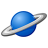# QlikView App Dev

Discussion Board for collaboration related to QlikView App Development.

cancel
Showing results for
Did you mean:Creator II

## Set analysis with len( variable)

Guys,

I've built a set analysis for a textbox but i'm having troubles in checking a variable lenght.

sum( {<'\$(=vVariable)' = {"=len(\$(=vVariable)) =0"} >} DIMENSION)

Does anyone have an idea?

9 RepliesEmployee

Please add this formula as an expression to a straight table with no label on it

Mouse over the header and check how your expression is beeing evaluatedMVP

How you define here vVariable

Before develop something, think If placed (The Right information | To the right people | At the Right time | In the Right place | With the Right context)Creator II
Author

Hi Anil,

The vVariable here is a number.Creator II
Author

Everything seems right in this. but it is not working.Employee

Would you mind posting a screenshot of your test?Employee

vVariable returns a number?

'\$(=vVariable)' = {} it´s supposed to be a fieldname so you can use as a Set Analysis expressionCreator II
Author

Is there a way of putting a number on it?Creator II
Author

The function sum( {<'\$(=vVariable)' = {"=len(\$(=vVariable)) =0"} >} DIMENSION)

returns  =sum({<'' = {"len()"=0}>} DIMENSION)  when I set the variable's value null.

I think the set analysis cannot retrieve a value because it cannot evaluate a null value in the left part of equation (=sum({<'' = ).

Do you have an idea to overcome that?Employee

Let´s start from the beginning...

What´s your intention? Maybe undestanding what you trying to do we can help a little better

A set analysis expression is supposed to have :

Sum({<aField={"Some search expression"}>}Value)

You can have a variable on left side of expression since this variable returns a fieldname